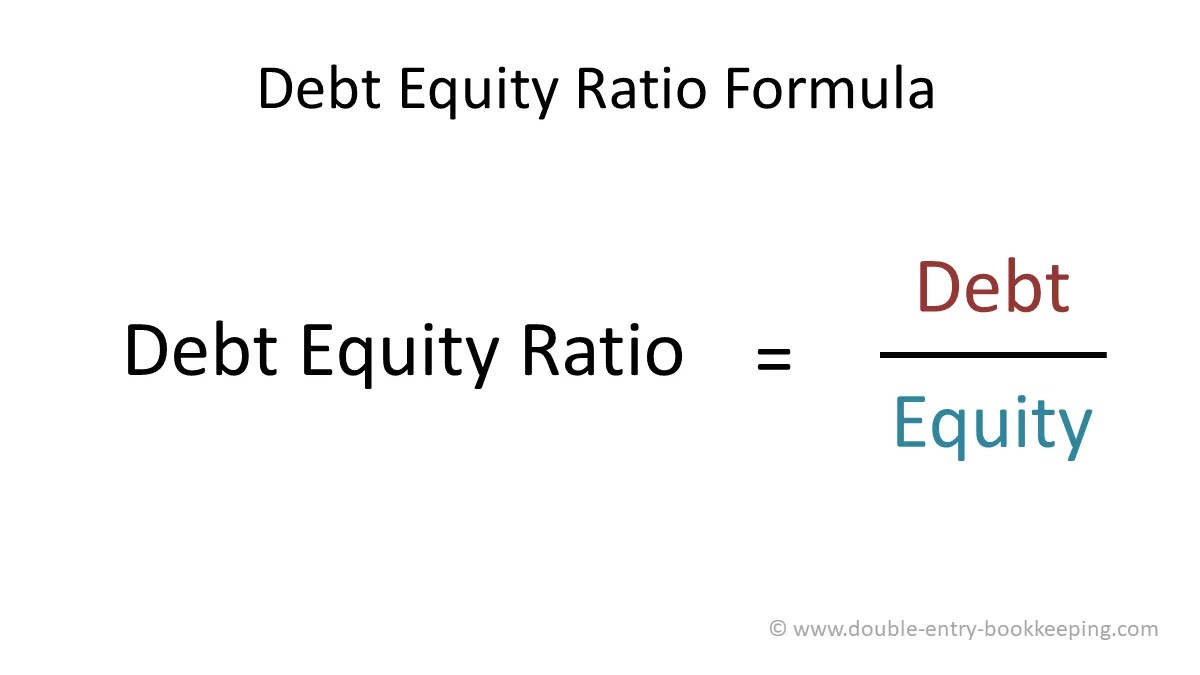Select Page

# Accounting Ratios- A beginner’s Guide

Why not start estimating simple accounting ratios before you regularly do all of the bookkeeping basics required for your company?

The accounting ratios are measured using figures listed on the balance sheet and income statement to provide you with important details, both now and in the future, which will help you develop your management.## What the Accounting Ratios are?

Although you might find it difficult initially, accounting ratios are easy estimates using the figures in the financial statements of your company.

Accounting ratios are not much of a problem to apply simple arithmetic logics to, these days software’s have replaced the old methods mostly.

Such a simple ratio can be used for the estimation of expected growth and for output comparisons (for example between different stores), and it can be very useful in finding support from investors or financial institutions.

Any company owner is able to execute basic accounting ratios, however ratio analysis can be difficult and a detailed task can best serve the CPA.

## The most profitable accounting ratios for independent businesses

The best accounting ratios for small businesses to track accounting rates differ between basic and complex. As a small business owner, you probably want to rely on the simplified ratios that give the organization and financial health useful statistics.

Some of the accounting ratios which can easily be calculated are given below.

### Percentage of Sales/Gross Profit Margin Ratio:

It is an essential ratio for minor businesses, which is not even difficult to calculate.

Take your gross profits and reduce the cost of the product sold using the figures from the income statement. This is then split into profits to reach the total margin of profit.

Let’s take an example, if your salary is $28,000, and your merchandise selling are$6,000, then your gross net income would be $22,000.Following the given values, calculation will be: 28,000 – 6,000 / 28,000 = 0.78 This means that you have a 78 percent gross profit margin, which is$0.78 with each dollar you sell.

### Profit Margin Ratio:

The profit margin ratio, equivalent to the ratio of gross profit margin, enables to understand the overall earnings of your company, rather than only prices of product sold, by cutting all expenses.

It offers you a strong idea of how well the company is doing overall after all costs have been incurred.

Your gross margin is determined using:Start with your 25,000 dollars sales to get your profit margin ratio. Subtract the net cost, which is 18,500 dollars and leaves 6,500 dollars in total. Then split your net profits up to your benefit margin by your total sales.

15,000 – 18,000 dollars = 6,500 dollars / 25,000 dollars = 0.026

A 25% profit margin or higher is a positive indication of a successful company.

### Quick ratio

Rapid ratio determines company’s capability to satisfy its imminent financial obligations. You collect fast ratio numbers from your account to compare your net asset to your total liabilities.

The method for calculating a rapid ratio:Your existing wealth would be 60,000 dollars if your company has an inventory of 90,000 dollars or 30,000 dollars. In order to achieve a fast percentage, you will then break this amount into your existing liabilities of 35,000 dollars.

90 thousand dollars – 30 thousand dollars = 500 thousand Dollars / 35 thousand = 1.71

This assumes that every $1.00 in liabilities you actually have$1,71 in cash. Businesses should maintain a 1-to-1 ratio, which ensures that you will have as many (or more as liabilities for properties.

### Debt-to-equity ratio

The ratio of corporate obligation and institutional interest, also known as the debt-to-asset ratio, is seen. It is a clear indication of how much debt, such as bonds, funds your corporate properties and how much equity is funded.

It is very easy to calculate a debt-to-equity ratio and use balance sheet figures.The debt-to-equity rate will be 0.69 to a firm with 1.25 million dollars in liabilities and 1.8 million dollars in equity.

125 thousand dollars / 180 thousand dollars = 0.69.

Any valuation that approaches 1 would mean that more interest is supported on the business properties, to be avoided if possible.

### A Tracker is set on your company using ratios:

Using easy averages is an excellent way to look over health of finance and results. They can even serve to signal that an organization can go the wrong way.

So, take the balance sheets and financial statements out and begin today to determine the company accounting ratios.

You will get surprised to know how simple it is.